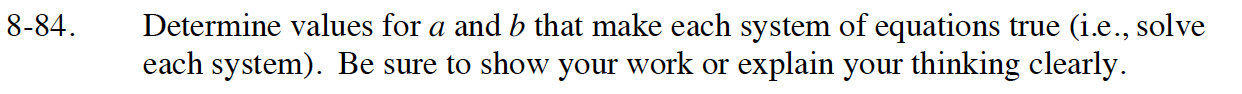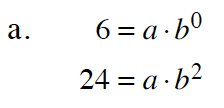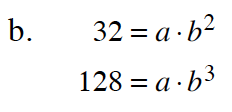### Home > INT1 > Chapter Ch8 > Lesson 8.1.6 > Problem8-84

8-84.
1. Determine values for a and b that make each system of equations true (i.e., solve each system). Be sure to show your work or explain your thinking clearly. Homework Help ✎

2.  a. 6 = a · b0 24 = a · b2 b. 32 = a · b2 128 = a · b3What is b0 equivalent to?

Once you have the value for a use it to solve for b in second equation.Solve both equations for a.

Set both equations equal to each other (Equal Values Method), and multiply both sides by the common denominator of both fractions.

Solve for b and substitute b back into one of the original equations to solve for a.

a = 2, b = 4